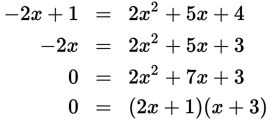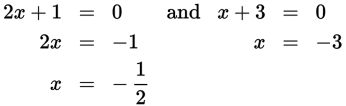# SAT Math Multiple Choice Question 336: Answer and Explanation

### Test Information

Question: 336

6. At what value(s) of x do the graphs of y = -2x + 1 and y = 2x2 + 5x + 4 intersect?

• A.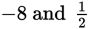• B.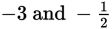• C.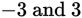• D.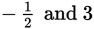Explanation:

B

Difficulty: Medium